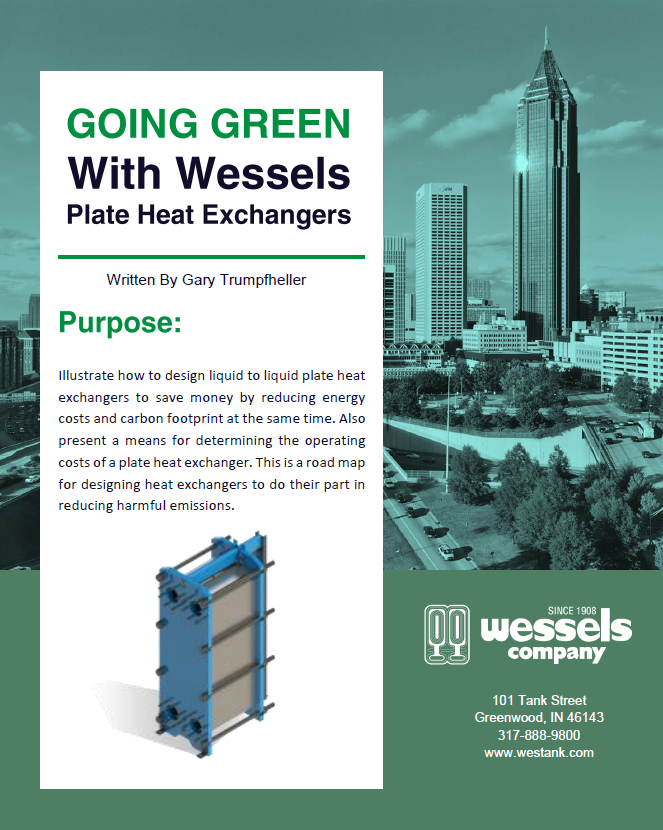#Download the Full White Paper “Going Green with Wessels Plate Heat Exchangers”

# Purpose:

Illustrate how to design liquid to liquid plate heat exchangers to save money by reducing energy costs and carbon footprint at the same time. Also present a means for determining the operating costs of a plate heat exchanger. This is a road map for designing heat exchangers to do their part in reducing harmful emissions.

# History:

### And A Changing Future

Plate heat exchangers are used for the transfer of heat or cold into or out of a commercial building. They have a very efficient design transferring 100% of the energy from one fluid to the other. They are a vital component for providing economical chilled water for air conditioning to residential and office buildings, hospitals, and especially data centers where they help to speed up and reduce the cost of millions of high-speed data transactions. Plate heat exchangers are also utilized for ground and air source heat pumps and large city or college district heating/cooling systems that save energy and reduce cost along with their carbon footprint.

For too many years, most HVAC projects have been driven by “lowest installed cost” instead of future savings.

For too many years, most HVAC projects have been driven by “lowest installed cost” instead of future savings. That is especially true in heat exchanger design. The size and cost of a plate heat exchanger is driven by its heat transfer coefficient, which is the rate of the transfer of heat from one liquid to another. The heat transfer coefficient is increased significantly by making the corrugations on the plates more resistant to flow causing a more turbulent flow pattern. This reduces the size and cost of the heat exchanger, but the energy cost increases due to the greater pump horsepower required to overcome this increased resistance.

In heat exchanger design, if not specified, the heat transfer engineer will use a maximum pressure drop of 10 psi to yield the highest heat transfer coefficient and lowest Installed cost heat exchanger possible. This direction is short sighted! To go green, we must think about future long-term benefits.

# Details:

### Crunching the Numbers

To first determine the operating cost of an HVAC plate heat exchanger based on the water flow and pressure drop through the heat exchanger:

###### 1)      Calculate the Horsepower (HP) required to move the fluid through the heat exchanger:

HP = GPM X FT X SG / 3960

Where: GPM = water flow in Gallons Per Minute through the heat exchanger

FT = head loss in Feet through the heat exchanger

SG = Specific Gravity of the fluid (1.0 for water)

3960 = 33,000 ft-lb/min over 8.33 lb/gallon

###### 2)      Calculate the Motor Horsepower (MHP) required:

MHP = HP / (e1 X e2) = Motor Horsepower

Where: HP = horsepower

E1 = motor efficiency. Assume 0.95

E2 = pump efficiency. Assume 0.80

###### 3)      Calculate the Kilowatt usage of the heat exchanger based on flow and pressure drop:

Where: KW = 0.745 X MHP = KiloWatts = Energy consumption

KW = (GPM X FT X SG) / 3960 / ((e1 X e2) X 0.745

KW = GPM X FT /3881

Note: This is the power required to move water through the heat exchanger only.

It does not include other system effects.

###### 4)      Calculate the annual operating cost of the heat exchanger:

Where: AOC = Annual Operating Costs = \$ / year

If utility cost = \$0.10 / kwh and there are 6000 hours per year of operation the
Annual Operating Cost of the heat exchanger becomes:

AOC = GPM X FT / (3881 X \$0.10 kwh X 6000 hours/year)

AOC = GPM X FT X \$0.15 = \$ / year

# Calculate:

A typical application is to cool 2000 gpm of water from 55 ⁰F to 45 ⁰F using 2000 gpm of water at 43 ⁰F on the other side. This requires a total heat transfer of 10,000,000 btu/hr.

Using a 23 ft (Approximately 10 psi) of pressure drop, Wessels sizing software calculates the following:

AWP83 with 315 plates and 3892 square feet of surface area at a cost of \$52,000

The Annual Operating Cost = (2,000 gpm)( 20 ft )(\$0.15) = \$6,000/yr

Using a 12 ft (Approximately 5 psi) of pressure drop, Wessels sizing software calculates the following:

AWP83 with 341 plates and 4216 square feet of surface area at a cost of \$57,000

The Annual Operating Cost = (2,000 gpm)( 10 ft )(\$0.15) = \$3,000/yr

# Final Thoughts:

### Going Green Means Saving Green

In the previous example, the old idea of using a maximum pressure drop of 10 psi results in a slightly lower installed cost of \$52,000 versus \$57,000.

However, if your goal is to be more energy efficient and save money in the long term, then taking the 5 psi selection that operates at \$3,000/yr versus the \$6,000/yr of the
10 psi selection cuts the energy consumption and annual operating cost in half. Doing a very simple pay back calculation, the larger heat exchanger’s energy efficiency results in a 1.7-year return.

To be energy conscious with heat energy and an eye to the future, the conscientious engineer should always specify lower pressure drops through heat exchangers to save energy, reduce carbon emissions, and make a greener future for everyone.

All Wessels plate and frame heat exchangers are AHRI certified Standard 400 to guarantee efficient performance

Download the Full White Paper “Going Green with Wessels Plate Heat Exchangers”